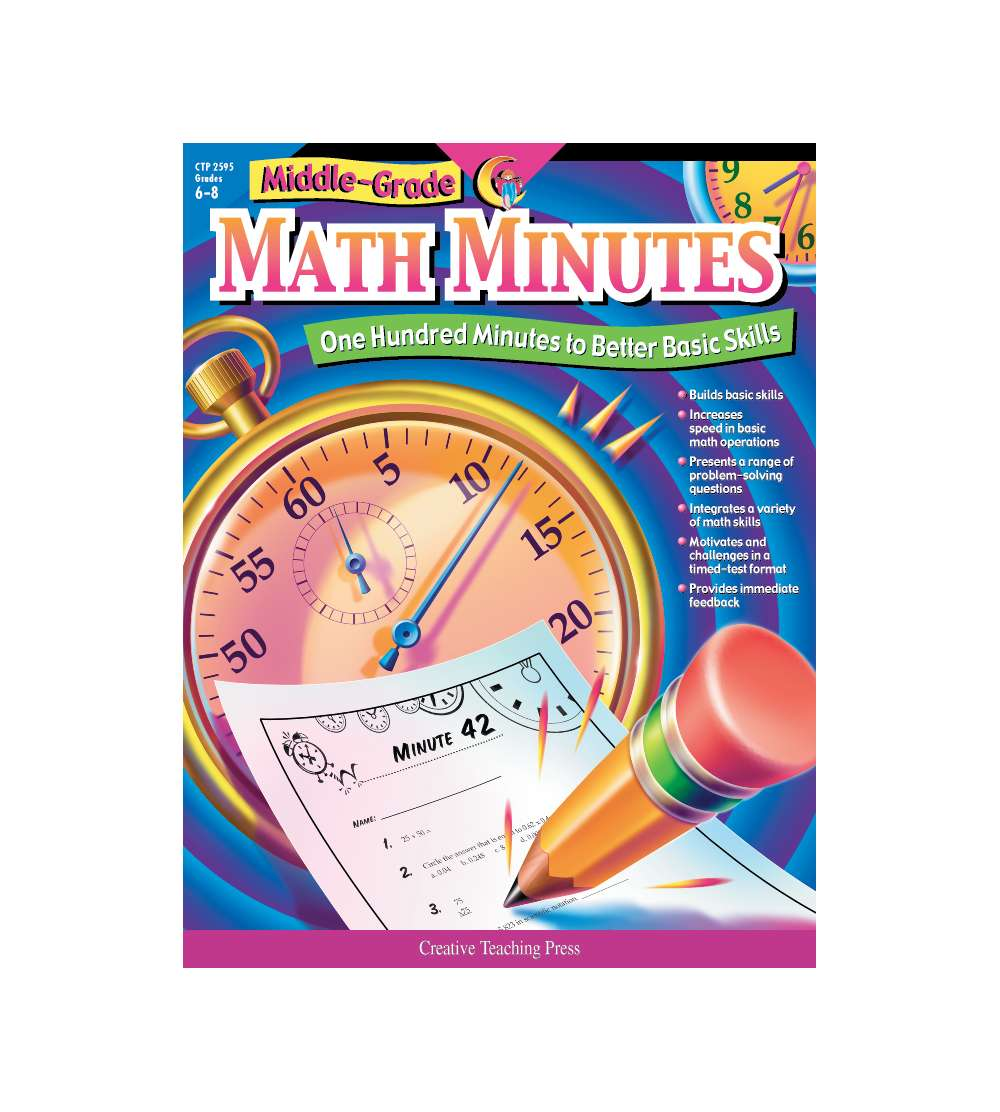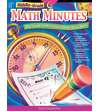•### Product Media

Product Number: CTP2595
\$16.99
Middle-Grade Minutes features 100 "Minutes" to help students build basic skills, increase speed in basic math operations, and...

Middle-Grade Minutes features 100 "Minutes" to help students build basic skills, increase speed in basic math operations, and integrate a variety of math skills. Each Minute consists of 10 classroom-tested problems of varying degrees of difficulty, integrating problem solving and basic math skills. Students become active learners as they discover mathematical relationships and apply acquired understanding of complex situations and solutions to realistic problems.

Now there's a way for students to practice their math skills in a timed situation, enhance their overall math fluency, and have fun at the same time! Middle-Grade Math Minutes provides students with practice in every key area of middle-grade math instruction, including:

• basic multiplication and division facts
• money
• graphing
• problem-solving
• math vocabulary
• fractions
• geometric shapes
• probability
• estimation
• decimals
• measurement
• powers of ten
• square roots
• percents
• multiples and factors
• and more!

Middle-Grade Math Minutes features 100 "Minutes." Each Minute consists of ten classroom-tested problems of varying degrees of difficulty, integrating problem solving and basic math skills. This unique format offers students an ongoing opportunity to improve their own fluency in a manageable, nonthreatening format. The quick, one-minute format combined with instant feedback makes this challenging and motivational assignment students will look forward to each day. Students become active learners as they discover mathematical relationships and apply acquired understanding to complex situations and to the solution of realistic problems in each Minute.

CTP2595